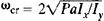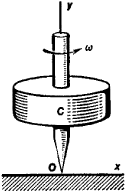# Stability of Motion

The following article is from The Great Soviet Encyclopedia (1979). It might be outdated or ideologically biased.

## Stability of Motion

one of the most important concepts in mechanics. The motion of any mechanical system, such as a machine, gyroscopic device, aircraft, or missile, is dependent on the forces at work and on the initial conditions, that is, on the positions and velocities of the points in the system at the moment when the motion is initiated. Given these forces and initial conditions, it is possible to calculate theoretically how the system will move. The motion thus calculated is called unperturbed. However, since all measurements are made only to some degree of accuracy, the true values of the initial conditions in practice will usually differ somewhat from the calculated values. In addition, a mechanical system may be subjected in the course of the motion to small, random influences that were not considered in calculations. This is also equivalent to a change in the initial conditions. Departures of the initial conditions from the calculated values that occur for various reasons are called initial perturbations, and the motion that the system will undergo with such perturbations present is called the perturbed motion.

The effect of initial perturbations on the characteristics of a system’s motion, such as the trajectories and velocities of the system’s points, may be twofold. With fairly small initial perturbations, if any of the characteristics differs very little from the value they should have for unperturbed motion continuing ad infinitum, the motion of the system with respect to such characteristics is termed stable. However, with initial perturbations that are arbitrarily small but not zero, if a characteristic differs more and more from the value it should have for unperturbed motion, the motion of the system with respect to the characteristic is termed unstable. These definitions correspond to A. M. Liapunov’s definition of stable motion. The conditions for which the motion of a mechanical system is stable are called stability criteria.

As an example, we will discuss a gyroscope that has a vertical axis and that rotates about this axis with an angular velocity ω (Figure 1). Theoretically the axis of the gyroscope ought to remain vertical for any value of ω in practice, however, when ω is less than a certain value ωcr, with any small perturbation the axis will deviate more and more from the vertical. If ω is greater than ωcr, small perturbations cause practically no change in the direction of the axis. Consequently, when ω < ωcr, the gyroscope is unstable with respect to the direction of its axis; it is stable when ω > ωcr. The latter inequality is the stability criterion. Herewhere P is the weight of the gyroscope, a is the distance from the point of support O to the center of gravity C, and Ix and Iy are the moments of inertia of the gyroscope relative to the x and y axes, respectively.Figure 1

The theory of stability of motion is of great practical importance in many fields of technology, inasmuch as various types of engines, motor vehicles, aircraft, rockets, gyroscopic devices, and automatic control systems should exhibit stability of motion. The problem of stability of motion arises in celestial mechanics in connection with studies of how long the structure of the solar system will be maintained and in research on binary stars.

### REFERENCES

Chetaev, N. G. Ustoichivost’ dvizheniia, 2nd ed. Moscow, 1955.
Duboshin, G. N. Osnovy teorii ustoichivosti dvizheniia. [Moscow] 1952.
Krasovskii, N. N. Nekotorye zadachi teorii ustoichivosti dvizheniia. Moscow, 1959.
Malkin, I. G. Teoriia ustoichivosti dvizheniia. Moscow-Leningrad, 1952.
Merkin, D. R. Vvedenie v teoriiu ustoichivosti dvizheniia. Moscow, 1971. (Contains bibliography.)
References in periodicals archive ?
Dynamics and Stability of Motion of Shock and Hybrid Systems
According to Osipenko , in his excellent paper titled "Center Manifolds", Lyapunov , in his dissertation "General Problem on Stability of Motion," was perhaps one of the pioneers in the study of the stability of dynamical systems, creating the foundations of the modern theory of the stability in dynamical systems.
Lyapunov, The general problem of the stability of motion (in Russian) [Phd.
The table of each stepping motor moves (motion 10+0.5 mm) in rolling ways, which secure a high stability of motion and slight frictional force.
Gyorgrey, "On the non-linear stability of motion's around L5 in the elliptic restricted problem of the three bodies," Celestial Mechanics and Dynamical Astronomy, vol.
After preliminaries, they cover analyzing the boundedness of motion, analyzing the stability of motion, the stability of weakly perturbed systems, and the stability of systems in Banach spaces.
The general problem of stability of motion. Gostekhizdat, 1950; Princeton Univ.
of California at Los Angeles department of engineering, addresses the fundamentals of kinematics, rigid body dynamics, linear control theory, orbital environmental effects, and introductory theory of the stability of motion. Theory is supplemented by examples of actual spacecraft and description of spin, dual-spin, three axis active, reaction wheel, control movement gyro, gravity gradient, and magnetic control systems.
Week 7: The asymmetric free top, stability of motion about axes, the Poinsot sphere
Stability of motion; the role of multicomponent Liapunov's functions.

Site: Follow: Share:
Open / Close